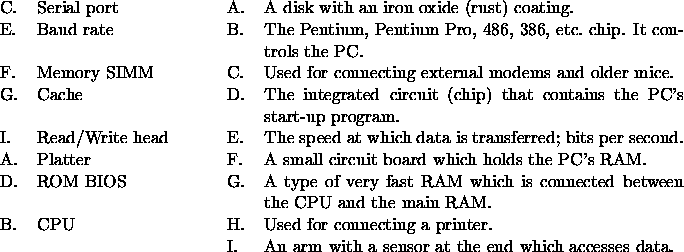# Quiz 2 Solution

CS 14A

60 pts., Feb. 28

1. (8 pts.) Match each of the following terms to their definitions:(There is one fewer item in the left column then there is in the right column.)

2. (4 pts.) Which of the following PC characteristics would have to be checked in order to determine if a software package could be run? (Circle all that apply.)
1. The family to which the processor chip belongs. (Circle)
2. The amount of cache.
3. The amount of RAM. (Circle)
4. The amount of free hard disk space. (Circle)
5. The speed of the network card.
6. The size of the monitor.

3. (4 pts.) The first customer who purchased a commercial computer was
1. IBM.
2. ABC.
4. Univac.
5. The Pentagon.
6. None of the above.

4. (4 pts.) Which of the following was a programming language designed for business processing?
1. FORTRAN.
3. BASIC.
4. Machine code.
5. None of the above.

5. (4 pts.) Who of the following was involved in the development of ENIAC?
2. Turing.
3. Shockley.
4. Kilby.
5. None of the above.

6. (4 pts.) Which type of printer has the highest print quality?
1. Dot matrix.
2. Ink jet.
4. Thermal wax.
5. None of the above.

7. (4 pts.) Integrated circuits were introduced during which computer generation?
1. The first.
2. The second.
4. The fourth.
5. The fifth.

8. (4 pts.) Which of the following is an editing step?
1. Word wrap.
2. Justification.
4. Boldfacing.
5. None of the above.

9. (4 pts.) When a graphic moves with a paragraph in a document it is
2. framed.
3. cropped.
4. formatted.
5. None of the above.

10. (6 pts.) Give three reasons why a word processor is an improvement over a typewriter.

Ability to format text within a document; Ability to save a document for recall later; Ability to edit a document.

11. (2 pts.) What is ASCII used for?

A code for storing character data in memory or storage.

12. (4 pts.) Describe the Turing test.

A test of machine intelligence. A ``judge'' communicated remotely with a computer and a person (not knowing which was which). The judge had to determine which conversant was which, through asking questions and analyzing responses.

13. (4 pts.) In the document production process, what is editing?

The act of revising a document.

14. (4 pts.) What is word wrap?

The feature which automatically brings a word down from one line in which it extends past the right margin to the beginning of the next line.

Thomas P. Kelliher
Tue Mar 4 12:28:38 EST 1997
Tom Kelliher